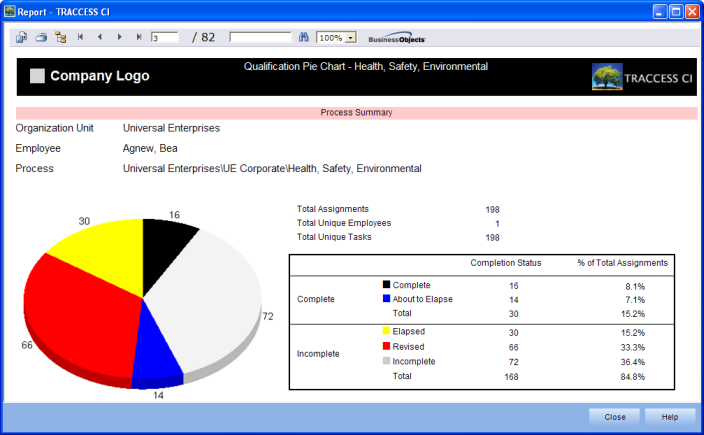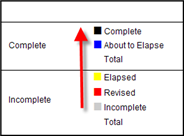The Qualification Pie Chart Report shows a visual representation of the qualifications, without all of the details found in the Qualification Report.The Qualification Pie Chart shows a breakdown of the statuses of selected Org Unit's or Learners' Tasks in the system.  This breakdown represents a roll-up of the entire Task, as opposed to showing the status of each of the Task components.

The overall status of a task is often simple to figure out:

• K only = Complete --> Overall = Complete

• K = Incomplete, C = Incomplete --> Overall = Incomplete

However, to determine the overall status of a Task that contains two components is determined by following an "Order of Precedence".  This order of precedence is as follows:

• Incomplete - either half (or both) are incomplete

• Revised - either half (or both) are revised (without being incomplete)

• Elapsed - either half (or both) are elapsed (without being incomplete or revised)

• About to Elapse - either half (or both) are about to elapse (without being incomplete, revised or elapsed)

• Complete - both halves are complete (without being incomplete, revised, elapsed or about to elapse).

For example:

• K = Complete, C = About to Elapse --> Overall = About to Elapse

• K = Revised, C = About to Elapse --> Overall = Revised

• K = Elapsed, C = Revised --> Overall = Revised

• K = About to Elapsed, C = Elapsed --> Overall = Elapsed

• K = Incomplete, C = Revised --> Overall = Incomplete

Please Note:  Since it may be difficult to remember the order of precedence, try working up the legend of statuses starting at Incomplete.  If any component of a Task is Incomplete, it will be represented as Incomplete (White).  If any component of a task is Revised, (and the other component is anything but Incomplete), the Task will be represented as Revised (Red).Since an overall status of Incomplete will be far more common than any other status, the Qualification Pie Chart will likely not display all of the colors, all of the time.  The above graphic is just a representation of how these colors would appear.